# LOG#065. SM(VII): phenomenology.

##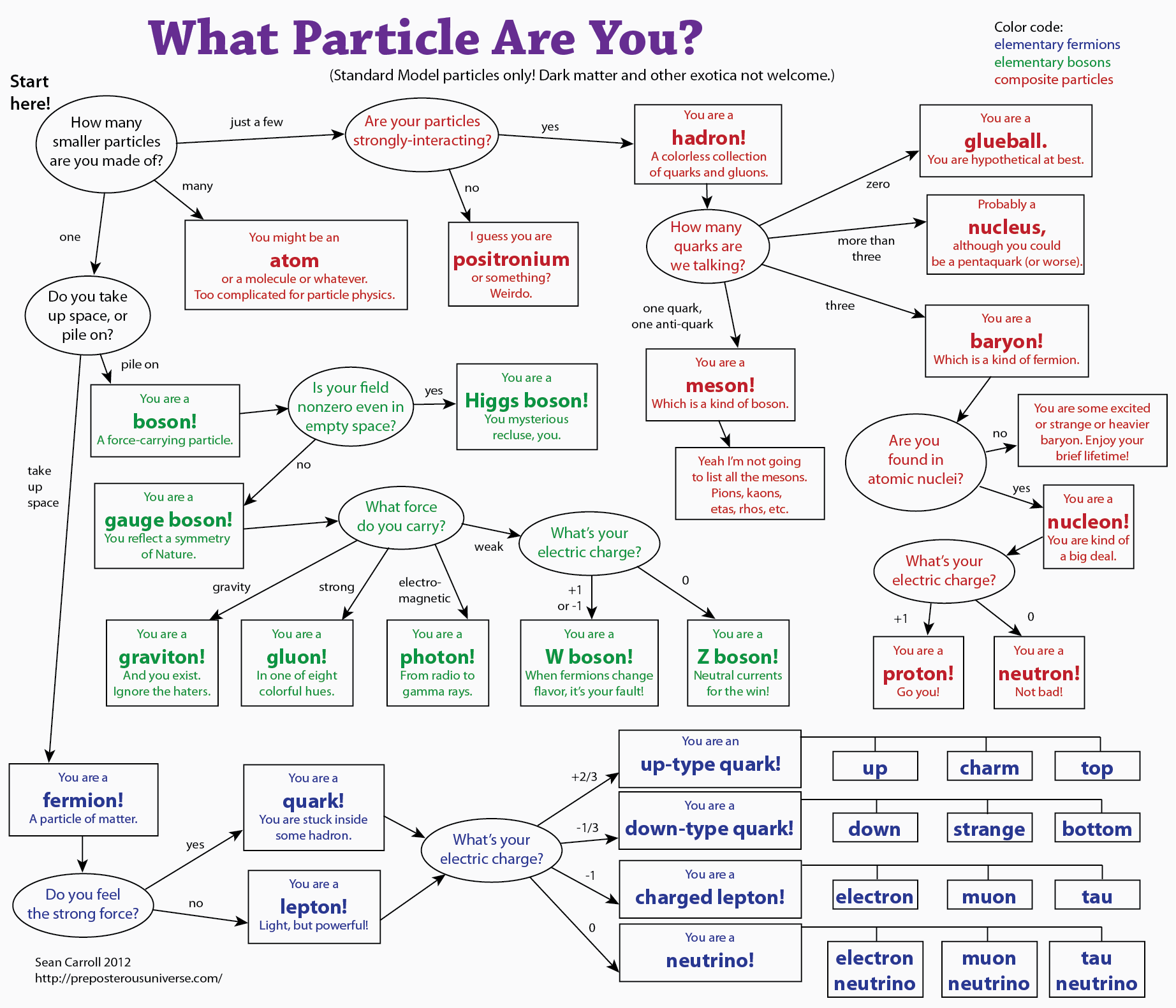The above picture is a cool mind map by the cosmologist and particle physicist Sean Carroll. It summaries somehow the phenomenological charges of the Standard Model plus the gravitational sector we do not know at quantum level.

## Physical Higgs sector

The Higgs field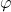is a field with TWO complex components, or equivalently if you want, FOUR real components in a suitable basis. It has 4 total degrees of freedom (DOF) from the real viewpoint. Three of these DOF are used in the SSB mechanism of mass generation via the gauge fields. Sometimes we say that those 3 DOF are “eaten up” by the Goldstone bosons. The final result is that we have a real scalar field! And the vacuum expectation value (or v.e.v.) is not zero! Then, we can write the Higgs field in the unitary gauge to be: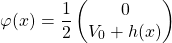where we have defined the physical Higgs field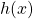and where we have. The quantum excitations of the Higgs field are called the Higgs bosons. These particles are predicted by the SM, so we have to find them experimentally. In this year, one ( if not the most) of the most important achievements is the finding of the Higgs boson by the LHC. It was reported by the LHC, during the summer, and it was confirmed independently by the two main collaborations of this wonderful machine/collider: ATLAS and CMS. It deserves the Nobel Prize such a discovery but I will not tell him my opinion about who and when are going to win the prize, or who or when should win it.

By the other hand, using the above equation for the physical Higgs field, we can rewrite the Yukawa pieces into “physical Yukawa pieces”:The coupling constant is, thus, proportional to the fermion mass through the physical Higgs field! We can make the following split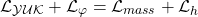We would obtain the fermion mass terms as follows:This lagrangian corresponds to the so-called Higgs sector of the SM. The physical Higgs field is massive and it interacts with itself and with the massive weak interacting bosons, i.e., it only interacts with the Z and W bosons. If you want to put it in words, the Higgs field is “transparent” for the EM photon field and the QCD gluon fields but it acts like some kind of nonlinear medium with the remaining (and massive) electroweak gauge bosons, and for the remaining fermions fields as well. That is, fermion fields feel the Higgs field and acquire mass as well via the SSB-Higgs mechanism. Some tree level Higgs diagrams are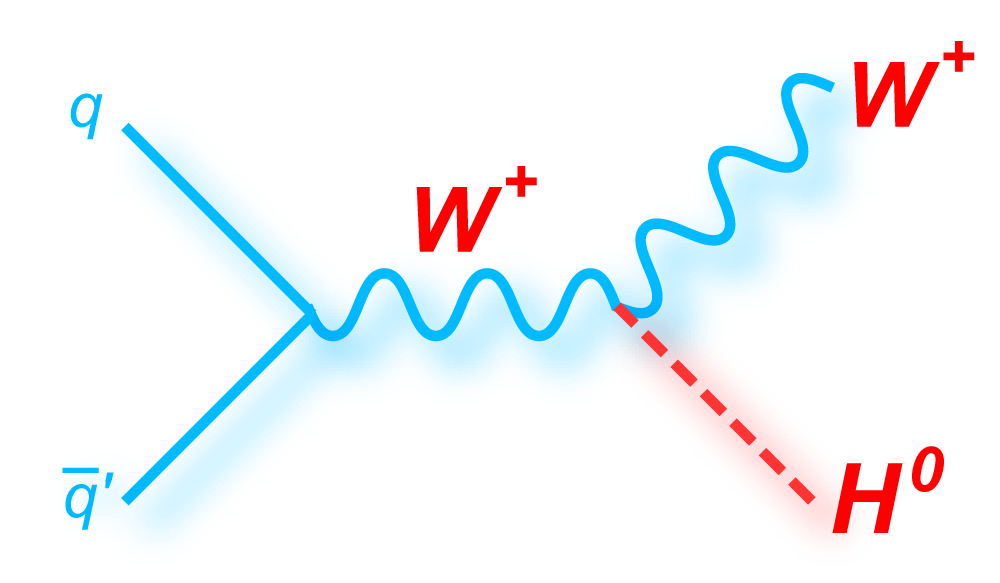The above two diagrams were important in Tevatron, the proton-antiproton Fermilab collider. Moreover, we also have, e.g., the interaction vertices: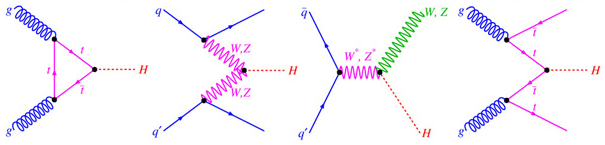## Fermion mass matrices

The Yukawa interaction in the unitary gauge becomesorand where we have written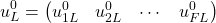This vector is a F-component array (column vector), and we have an analogue expression for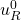. By the other hand,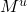is a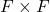fermion mass matrix: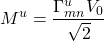It is induced by SSB ( Spontaneous Symmetry Breaking) andis the so-called Yukawa coupling matrix.

Remark: The Yukawa coupling matrix express fermion mass terms as functions of the physical higgs mass and its corresponging Higgs-fermion coupling, or equivalently, the Yukawa coupling matrix is related to the Higgs v.e.v. and/or the higgs-coupling and the electroweak boson mass. A truly remarkable fact!

Remark(II): In general the Yukawa coupling matrix is NOT diagonal, hermitian or even symmetric! To identify the physical particle content it is necessary to diagonalize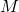by some unitary transformationsand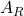on both sides (right and left) and onto the left-handed and right-handed fermion fields. In the special case thatis chosen to be hermitian, we can takeand thusis a completely diagonal matrix with eigenvalues equal to the physical masses of the quark tripletwith charge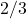. Similarly, we can perform the following transformations: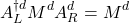The mass eigenstates are defined via unitary matrices as: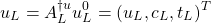and similarly for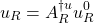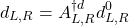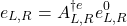In terms of the charged leptonic unitary transformation, we can chooseIndeed, we define the neutrino fields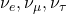as the weak interaction partners of the lepton triplet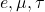.

## Higgs-Fermion couplings

The Higgs-fermion interactions are characterized by the following lagrangian piece:The Higgs-fermion coupling has some interesting features:

1) The coupling of the physical Higgs boson to the ith fermion is given by a coefficient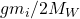. It is very small in general, and it is only “large” for the top quark.

2) The coupling Higgs-fermion is diagonal in the “flavor”-space: there is just one and only one Yukawa matris for every type of fermion, so the mass and the Yukawa matrices are diagonalized by the same transformations.

## CKM matrix: W-fermion interaction vertices

The W-bosons interact with fermions via weak interaction, through a lagrangian called “charged current” (CC) lagrangian: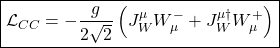The “weak charge-raising current” can be showed to be: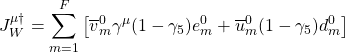or equivalentlyIn fact, this structure for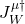owns what theoretical physicists call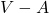, (vector-axial vector, or vector-pseudovector), form. This structure is essential in order to provide an explanation of parity violation experiments, charge violation experiments and CP violations. Indeed, the CP violations in the EW sector  are known to be maximally violated experimentally. The mismatch between unitary transformations for those states that relate the weak and the mass eigenstates for the up-down quark types lead to the existence of theunitary matrixin the above weak current. This is the CKM (Cabibbo-Kobayashi-Maskawa) or quark mixing matrix matrix. Experimentally, it is known that the CKM is very close to the unit matrix. By the other hand, for instance, if we make V with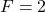, i.e., two families or generations, V is written aswith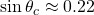, from experiments neglecting the 3rd family.is called the Cabibbo angle, and despite the fact of being two-flavored, the F=2 toy model provides a good approximation to the weak interactions of the quarks u, d, s, an c (up, down, strange, charm).

If we go to the complete F=3 case that is realized in the complete SM and, it seems, in Nature/the Physical Realm, we have the full F=3 CKM matrix given by:The matrix elements are generally complex numbers (it implies CP-violating “phases”!) and there are some common parametrization of the above matrix in the literature. I am not going to discuss mixing in detail here today, so you will have to wait if you want to see me how to explain quark and lepton mixing, and the structure hidden in their “oscillations”. In fact, we do know that there is a leptonic mixing analogue of the CKM matrix, the PMNS (Pontecorvo-Maki-Nakagawa-Sakata) matrix, and that matrix MUST be included in the weak current as well! It is an inevitable consequence of the structure of the SM lagrangian AND the phenomenological fact that neutral hadrons and neutrinos do oscillate!

This charged current weak interaction allow to mimic and old theory for weak interactions as an effective theory or just the so-called Fermi theory is a particular case of the (electro)weak interaction! In the limit of low transferred momentum, i.e., when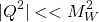the momentum term in the gauge W-boson physical propagetor can be neglected, and it leads to an effective zero-range (four-fermion field theory) interaction: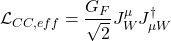And there, we can identify and relate the Fermi constant with the weak coupling constant and the W-boson mass:Fermi theory of the weak interaction is “derived” as an approximate or effective (today the preferred term is “emergent”) theory from the SM in the limit of small momentum transfer! Such the power of the SM and the electroweak unification is…

## QED and EM currents

QED or Quantum ElectroDynamics is based on the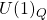subgroup that remains unbroken after SSB. The relevant sector of the SM is the lagrangian piece:We observe that the linear combination of the neutral gauge fields is just the usual photon field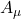that we know from Classical Electrodynamics! The electrical coupling is defined as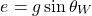where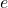is the electrical charge of the electron in absolute value. Moreover, we also have:The electromagnetic current is given by the following term: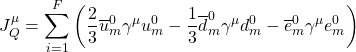or equivalentlyNote that it has “the same aspect” when we write the current in terms of weak or mass eigenstates! It is due to the fact that every SM fermion mix with each other in such a way they have the same electrical charge! Therefore, the electromagnetic current is automatically flavor-diagonal. We can say that electromagnetism is flavor blind or that flavor is electromagnetically “transparent”. I know these words are not “standard” but I think they express clearly the concept behind those equations.

## Neutral currents

In addition to the electromagnetic part, an extra piece arises in the EW sector in the SM lagrangian. It is:There, the linear combination of neutral fields is not the usual photon we know but a new massive Z boson field. It was a formidable prediction in the EW theory. The field strength of this new field is generally rewritten in terms of new “couplings”:It follows fromand then, the neutral current (NC) is given by the next lagrangian part of the SM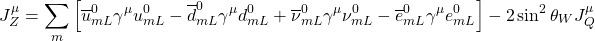or equivalently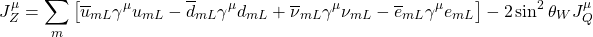Similarly to the electromagnetic current, the neutral current is flavor diagonal (i.e., flavor is “transparent” for neutral currents or neutral currents are flavor blind in the SM). Every SM fermion, thus, whenever they have the same charge and chirality, can “mix” with each other since they have the same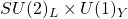charge assignments. This fact implies that the form of their currents/interactions are NOT affected by the unitary transformations that relate the mass and weak eigenstates. It is a deep conclusion whose consequences go beyond this introduction to the SM, but I promise to come back to the mixing issue in the near future.

Remark: The NC has two main contributions. The first one involves the left-chiral fields and it is purely V-A (vector-pseudovector) in form. The second one is proportional to the electromagnetic current with coefficient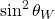and it is purely vector. Parity is violated in the neutral current interaction but it is NOT maximally violated. I could speak more about this topic (C,P, CP, T and likely CPT violations) in some additional post. But, you have to follow me closely if you want to learn more!

Remark(II): When the interactions between fermions happen in the limit that the momentum transfer is small compared to the Z-boson mass,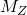, we can neglect theterm in the propagator and the interaction reduces to the Fermi theory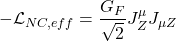The remarkable fact is that the coefficient is the same as in the charged current because we obtain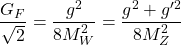This last consequence is caused by the folllowing reason: the difference in the Z couplings for the fermions compensates the difference in masses in the propagator.

This site uses Akismet to reduce spam. Learn how your comment data is processed.